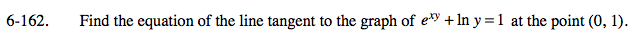### Home > CALC > Chapter 6 > Lesson 6.4.3 > Problem6-162

6-162.

Find the equation of the line tangent to the graph of exy + ln y = 1 at the point (0, 1). Homework Help ✎Use point slope form. You are given the point.

$\text{Differentiate to find the slope, }\frac{dy}{dx}.$

Evaluate at the given point.